# Kinematic Equations: List & Example

Lesson Transcript
Instructor: David Wood

David has taught Honors Physics, AP Physics, IB Physics and general science courses. He has a Masters in Education, and a Bachelors in Physics.

After watching this video, you will be able to explain what kinematics is, list the five most important kinematics equations, and use them to solve problems. A short quiz will follow. Updated: 07/24/2020

## What Is Kinematics?

Kinematics is the study of motion, without reference to the forces that cause that motion. Or in other words, kinematics focuses on position, velocity and acceleration, and doesn't deal with forces. In kinematics there are five important quantities: displacement (change in position), initial velocity, final velocity, acceleration, and time.

Initial velocity is how fast an object is moving at t = 0. Final velocity is how fast an object is moving when a time (t) is over. Displacement is how much the position changed by during that time (t). Acceleration is the rate at which the velocity changed during the time (t). And time is just...well, it's the time - the time that you're interested in, the time during which the object was moving, accelerating, or whatever.

In kinematics, we solve problems using graphs and equations. Today, we're going to focus on the equations.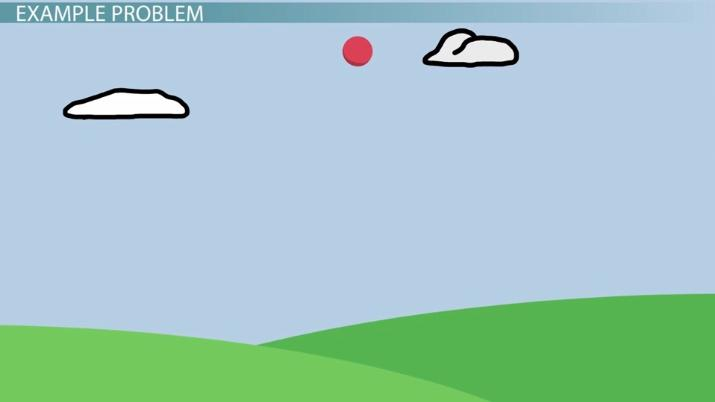An error occurred trying to load this video.

Try refreshing the page, or contact customer support.

Coming up next: Newton's First Law of Motion: Examples of the Effect of Force on Motion

### You're on a roll. Keep up the good work!

Replay
Your next lesson will play in 10 seconds
• 0:01 What is Kinematics?
• 1:09 Kinematics Equations
• 2:06 Example Problem
• 4:04 Lesson Summary
Save Save

Want to watch this again later?

Timeline
Autoplay
Autoplay
Speed Speed

## Kinematics Equations

There are five main kinematics equations you need to know about to solve problems.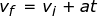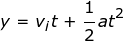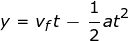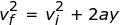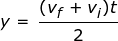In these five equations:

• t is the time measured in seconds
• vi is the initial velocity measured in meters per second
• vf is the final velocity measured in meters per second
• a is the acceleration, measured in meters per second squared
• y (or sometimes x) is the displacement, measured in meters

It's also important to note that for falling objects, the acceleration (a) is the acceleration due to gravity (g), which is always negative 9.8 meters per second squared.

Each of the five equations has four variables in it, with one variable missing. Whenever you're solving kinematics problems, you should be given three numbers, and asked to find a fourth. So all you have to do is find the equation with those four quantities in it, plug numbers in, and solve.

## Sample Motion Problem

Let's go through an example of how to use the equations. Let's say a ball is dropped from a height of 6 meters, and it falls until it reaches the ground. How long does it take to reach the ground?Well, first of all we should write down what we know. The displacement y is -6 meters. Why negative? Well, it's falling downwards. Usually in physics, we call upwards positive and downwards negative. This is rather arbitrary, though, and as long as all your signs are consistent, you should get the same answer.

Okay, now we have a potential problem: there are no other numbers in the question. But, the question tells us things that sneakily give us some other numbers we can use. For one thing the ball is falling, meaning falling under gravity. So the acceleration, as for all falling objects, is -9.8. Again, negative because the acceleration is pointed down.

And the question also tells us that the ball is dropped, which means that the initial velocity is zero. When you drop a ball, for the instant you let go of it, it isn't moving and its velocity is zero. And we're asked to find the time, t, so t = ?.

To unlock this lesson you must be a Study.com Member.

### Register to view this lesson

Are you a student or a teacher?